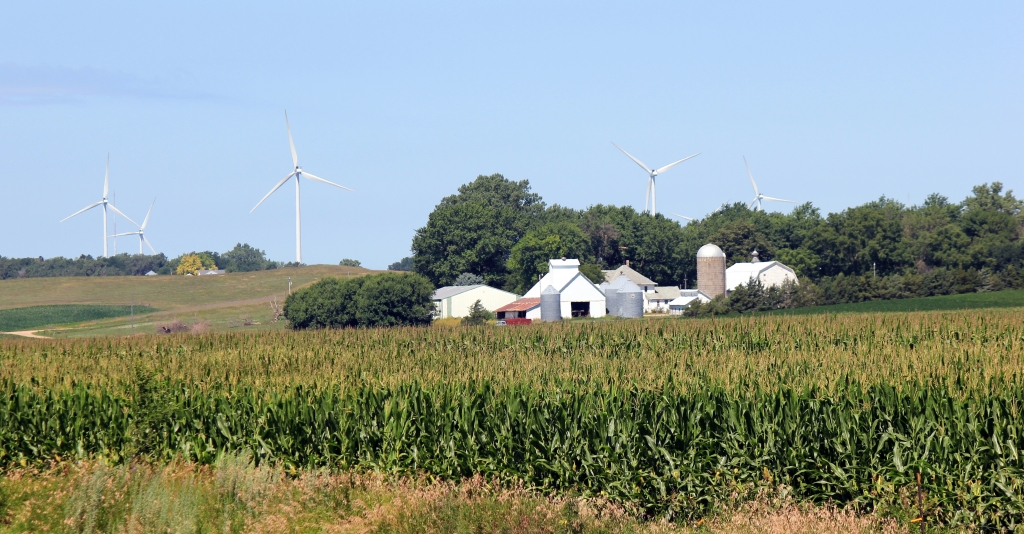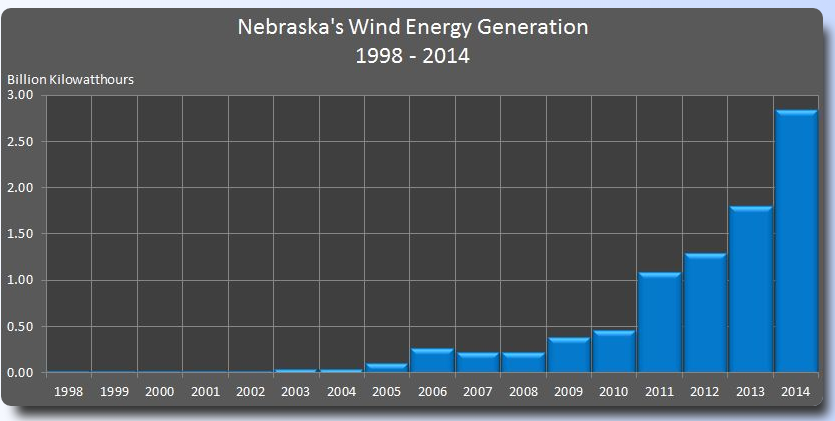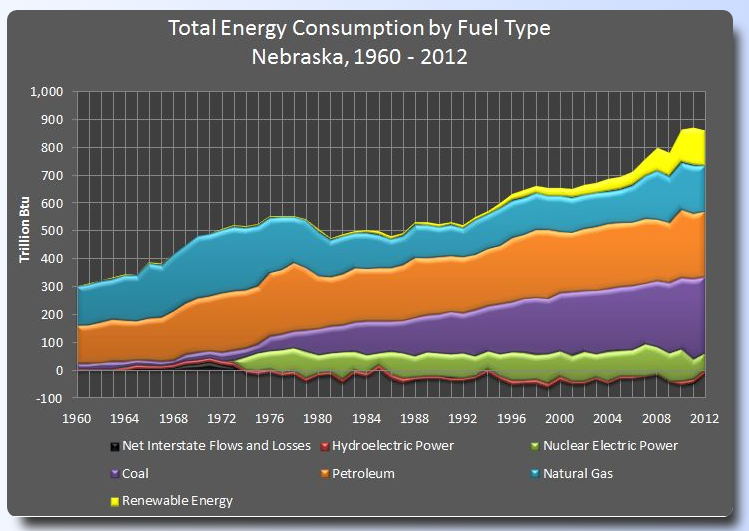President Obama announced his carbon pollution standards for power plants on 8/03/2015. In it he outlines a 32% cut in carbon dioxide emissions by 2030. Currently, Nebraska gets about 72% of its electricity from coal. According to the American Wind Energy Association, Nebraska has the potential of meeting more than 118 times the state’s current electrical needs using current wind energy technology.This begs the question, how is Nebraska doing with regard to renewable energy solutions?

According to the Nebraska Energy Office, wind energy generation has taken on an exponential growth type curve. Annual kilowatt hours generated for each year from 1998 to 2016 month-to-date are given in two tables.Use a graphing calculator, Excel, Geogebra, or Desmos to find the equation of the curve of best fit for the data. A caveat: graphing software likes easy numbers like 1 and 2 rather than 1998 and 1999 to work with when generating regression equations.

Next, use the Total Energy Consumption data to find the equation of the trend line for the total energy consumption in Nebraska for the same time frame. Be careful. A conversion between Btu and kWh is necessary.If wind energy generation continues to grow exponentially and energy consumption at a linear rate, in what year will wind energy production outpace total energy consumption in Nebraska?

Instructions for finding equations of best fit on using Desmos, Geogebra or a TI graphing calculator are linked and also available at Where do these equations come from?.

By | 2017-11-13T22:02:01+00:00 August 3rd, 2015|Graphing Calculator, PreCalculus|0 Comments

### About the Author: Stacie Bender